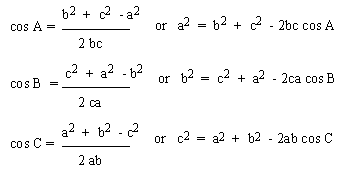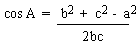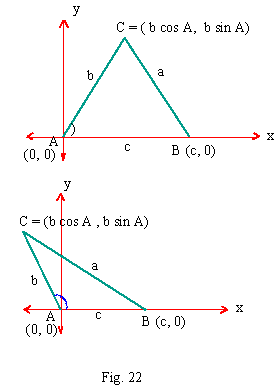Support the Monkey! Tell All your Friends and TeachersHome MonkeyNotes Printable Notes Digital Library Study Guides Study Smart Parents Tips College Planning Test Prep Fun Zone Help / FAQ How to Cite New Title Request

3.2 Law Of Cosines

If A, B and C are the measure of angles of a triangle ABC and a, b and c are lengths of sides opposite the angles A, B and C respectively, thenThese three formulas are called the Law of cosines or cosine rule. Let us place angle A of triangle ABC in standard position. Further Ð A < 1800 therefore point C is above x-axis. In two figures, Ð A is as acute and obtuse.

... AB = C \ B º (c, 0). Let C º (x, y). Since AC = b.

We have x = b cos A and y = b sin A

\ C º ( b cos A, b sin A). Now BC = a

By using distance formula

(BC)2 = (c - b cos A)2 + (b sin A)2

\ a2 = c2 - 2bc cos A + b2 cos2 A + b2 sin2 A

\ a2 = c2 - 2bc cos A + b2 (cos2 A + sin2 A)

\ a2 = c2 - 2bc cos A + b2 (1)

\ a2 = c2 - 2bc cos A + b2

\ a2 = b2 + c2 - 2bc cos A OR

\Your browser does not support the IFRAME tag.

Note that if A = 900 , then cos A = cos 900 = 0, giving a2 = b2 + c2 which is the "Pythagoras theorem" for right triangles. If the orientation of the triangle is changed to have B or C at the origin, then the other two versions of Law of cosines can be obtained.

Index

3. 1 Solving Right Triangles
3. 2 Law of Cosines
3. 3 Law of Sines
3. 4 The Ambiguous Case of Law of Sines
3. 5 Areas of Triangles
Supplementary Problems

Chapter 4Search: All Products Books Popular Music Classical Music Video DVD Toys & Games Electronics Software Tools & Hardware Outdoor Living Kitchen & Housewares Camera & Photo Cell Phones Keywords: